# An Introduction to XGBoost R package

## Introduction

XGBoost is a library designed and optimized for boosting trees algorithms. Gradient boosting trees model is originally proposed by Friedman et al. The underlying algorithm of XGBoost is similar, specifically it is an extension of the classic gbm algorithm. By employing multi-threads and imposing regularization, XGBoost is able to utilize more computational power and get more accurate prediction. Please refer to this tutorial for the details of the model.

One evidence of its accuracy is that XGBoost is used in more than half of the winning solutions in machine learning challenges hosted at Kaggle. We have prepared a (incomplete) list of winning solutions.

There’re various high-level interfaces. Currently there are interfaces of XGBoost in C++, R, python, Julia, Java and Scala. The core functions in XGBoost are implemented in C++, thus it is easy to share models among different interfaces. This post is going to focus on the R package `xgboost`, which has a friendly user interface and comprehensive documentation. Based on the statistics from the RStudio CRAN mirror, The package has been downloaded for more than 4,000 times in the last month.

The R package `xgboost` has won the 2016 John M. Chambers Statistical Software Award. From the very beginning of the work, our goal is to make a package which brings convenience and joy to the users. Thus we will introduce several details of the R pacakge `xgboost` that (we think) users would love to know.

## A 1-minute Beginner’s Guide

`xgboost` is available on both CRAN and Github. To install the stable/pre-compiled version from CRAN, simply run:

``````install.packages('xgboost')
``````

You can also install from our weekly updated drat repo:

``````install.packages("drat", repos="https://cran.rstudio.com")
install.packages("xgboost", repos="http://dmlc.ml/drat/", type="source")
``````

In order to run a machine learning algorithm, we need a data set first. `xgboost` has a demo data set about mushrooms. This data set records biological attributes of different mushroom species, and the target is to predict whether it is poisonous. Users can load the data with

``````require(xgboost)

data(agaricus.train, package='xgboost')
data(agaricus.test, package='xgboost')
train <- agaricus.train
test <- agaricus.test
``````

Each variable is a `list` containing two things, `label` and `data`. The next step is to feed this data to `xgboost`. Besides the data, we need to train the model with some other parameters:

• `nrounds`: the number of decision trees in the final model
• `objective`: the training objective to use, where “binary:logistic” means a binary classifier.

The simplest training command is as follows:

``````model <- xgboost(data = train\$data, label = train\$label,
nrounds = 2, objective = "binary:logistic")
``````
``````## 	train-error:0.000614
## 	train-error:0.001228
``````

We can make prediction on the test data set easily:

``````preds = predict(model, test\$data)
``````

Sometimes it is important to use cross-validation to exam our model. In `xgboost` we provide a function `xgb.cv` to do that. Basically users can just copy every thing from `xgboost`, and specify `nfold`:

``````cv.res <- xgb.cv(data = train\$data, label = train\$label, nfold = 5,
nrounds = 2, objective = "binary:logistic")
``````
``````## 	train-error:0.000921+0.000343	test-error:0.001228+0.000687
## 	train-error:0.001075+0.000172	test-error:0.001228+0.000687
``````

To get to know more details about the usage of `xgboost`, please visit our tutorial for more information.

## Efficient Algorithm

If you have experiences in training models on a large data set, then you probably agree that waiting for the training to be done is boring. Time is a resource, so the training speed of an learning algorithm is important. This is determined by both algorithm and implementation. We pay attention to these issues when building `xgboost`, thus we are confident that `xgboost` is one of the fastest learning algorithm of gradient boosting algorithm. The reasons for the good efficiency are:

• The computational part is implemented in C++.
• It can be multi-threaded on a single machine.
• It preprocesses the data before the training algorithm.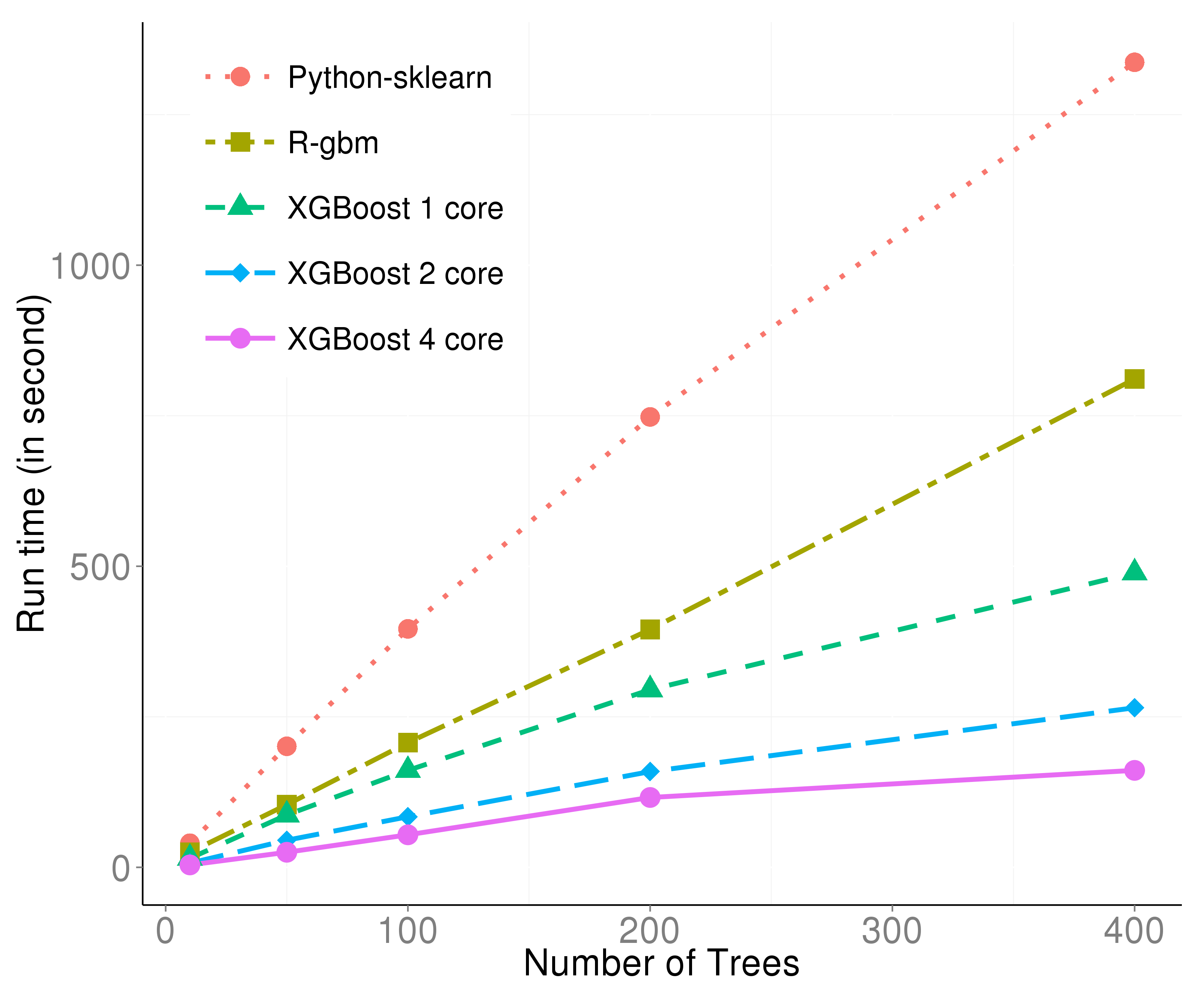This figure is generated with the dataset from the Higgs Boson Competition. It can be described from two aspects:

• With only one thread, the effect of preprocessing and C++ is already obvious.
• The multi-threading is almost linear with the number of threads, thus boosting the efficiency further.

## Convenient Interface

As the developers of `xgboost`, we are also heavy users of `xgboost`. We value the experience on this tool. During the development, we try to shape the package to be user-friendly. Here are several details we would like to share, please click the title to visit the sample code.

Customized Objective

`xgboost` can take customized objective. This means the model could be trained to optimize the objective defined by user. This is not often seen in other tools, since most of the algorithms are binded with a specific objective. With `xgboost`, one can train a model which maximize the work on the correct direction.

Let’s use log-likelihood as an demo. We define the following function to calculate the first and second order of gradient of the loss function:

``````loglossobj <- function(preds, dtrain) {
# dtrain is the internal format of the training data
# We extract the labels from the training data
labels <- getinfo(dtrain, "label")
# We compute the 1st and 2nd gradient, as grad and hess
preds <- 1/(1 + exp(-preds))
hess <- preds * (1 - preds)
# Return the result as a list
}
``````

Then we train the model as

``````model <- xgboost(data = train\$data, label = train\$label,
nrounds = 2, objective = loglossobj, eval_metric = "error")
``````
``````## 	train-error:0.001228
## 	train-error:0.001228
``````

The result should be equivalent to `objective = "binary:logistic"`.

Early Stopping

A usual scenario is when we are not sure how many trees we need, we will firstly try some numbers and check the result. If the number we try is too small, we need to make it larger; If the number is too large, we are wasting time to wait for the termination. By setting the parameter `early_stopping`, `xgboost` will terminate the training process if the performance is getting worse in the iteration.

``````bst <- xgb.cv(data = train\$data, label = train\$label, nfold = 5,
nrounds = 20, objective = "binary:logistic",
early.stop.round = 3, maximize = FALSE)
``````
``````## 	train-error:0.000921+0.000343	test-error:0.001228+0.000686
## 	train-error:0.001228+0.000172	test-error:0.001228+0.000686
## 	train-error:0.000653+0.000442	test-error:0.001075+0.000875
## 	train-error:0.000422+0.000416	test-error:0.000767+0.000940
## 	train-error:0.000192+0.000429	test-error:0.000460+0.001029
## 	train-error:0.000192+0.000429	test-error:0.000460+0.001029
## 	train-error:0.000000+0.000000	test-error:0.000000+0.000000
## 	train-error:0.000000+0.000000	test-error:0.000000+0.000000
## 	train-error:0.000000+0.000000	test-error:0.000000+0.000000
## 	train-error:0.000000+0.000000	test-error:0.000000+0.000000
## Stopping. Best iteration: 7
``````

Here we are doing cross validation. `early.stop.round = 3` means if the performance is not getting better for 3 steps, then the program will stop. `maximize = FALSE` means our goal is not to maximize the evaluation, where the default evaluation metric for binary classification is the classification error rate. We can see that even we ask the model to train 20 trees, it stopped after the performance is perfect.

Continue Training

Sometimes we might want to try to do 1000 iterations and check the result, then decide if we need another 1000 ones. Usually the second step could only be done by starting from the beginning, again. In `xgboost` users can continue the training on the previous model, thus the second step will cost you the time for the additional iterations only. The theoratical reason that we are capable to do this is because each tree is only trained based the prediction result of the previous trees. Once we get the prediction by the current trees, we can start to train the next one.

This feature involves with the internal data format of `xgboost`: `xgb.DMatrix`. An `xgb.DMatrix` object contains the features, target and other side informations, e.g. weights, missing values.

First, let us define an `xgb.DMatrix` object for this data:

``````dtrain <- xgb.DMatrix(train\$data, label = train\$label)
``````

Next we train the model with it:

``````model <- xgboost(data = dtrain, nrounds = 2, objective = "binary:logistic")
``````
``````## 	train-error:0.000614
## 	train-error:0.001228
``````

Note that we have the `label` included in the `dtrain` object. Then we make prediction on the current training data:

``````pred_train <- predict(model, dtrain, outputmargin=TRUE)
``````

Here the parameter `outputmargin` indicates that we don’t need a logistic transformation of the result.

Finally we put the previous prediction result as an additional information to the object `dtrain`, so that the training algorithm knows where to start.

``````setinfo(dtrain, "base_margin", pred_train)
``````
``````##  TRUE
``````

Now observe how is the result changed:

``````model <- xgboost(data = dtrain, nrounds = 2, objective = "binary:logistic")
``````
``````## 	train-error:0.000614
## 	train-error:0.000614
``````

Handle Missing Values

Missing value is commonly seen in real-world data sets. Handling missing values has no rule to apply to all cases, since there could be various reasons for the values to be missing. In `xgboost` we choose a soft way to handle missing values. When using a feature with missing values to do splitting, `xgboost` will assign a direction to the missing values instead of a numerical value. Specifically, `xgboost` guides all the data points with missing values to the left and right respectively, then choose the direction with a higher gain with regard to the objective.

To enable this feature, simply set the parameter `missing` to mark the missing value label. To demonstrate it, we can manually make a dataset with missing values.

``````dat <- matrix(rnorm(128), 64, 2)
label <- sample(0:1, nrow(dat), replace = TRUE)
for (i in 1:nrow(dat)) {
ind <- sample(2, 1)
dat[i, ind] <- NA
}
``````

Then we only need to specify the missing value marker, `NA`, in our code:

``````model <- xgboost(data = dat, label = label, missing = NA,
nrounds = 2, objective = "binary:logistic")
``````
``````## 	train-error:0.281250
## 	train-error:0.281250
``````

Practically, the default value of `missing` is exactly `NA`, therefore we don’t even need to specify it in a standard case.

## Model Inspection

The model used by `xgboost` is gradient boosting trees, therefore a model usually contains multiple tree models. A typical ensemble of two trees looks like this:

``````bst <- xgboost(data = train\$data, label = train\$label, max.depth = 2,
eta = 1, nthread = 2, nround = 2, objective = "binary:logistic")
xgb.plot.tree(feature_names = agaricus.train\$data@Dimnames[], model = bst)
``````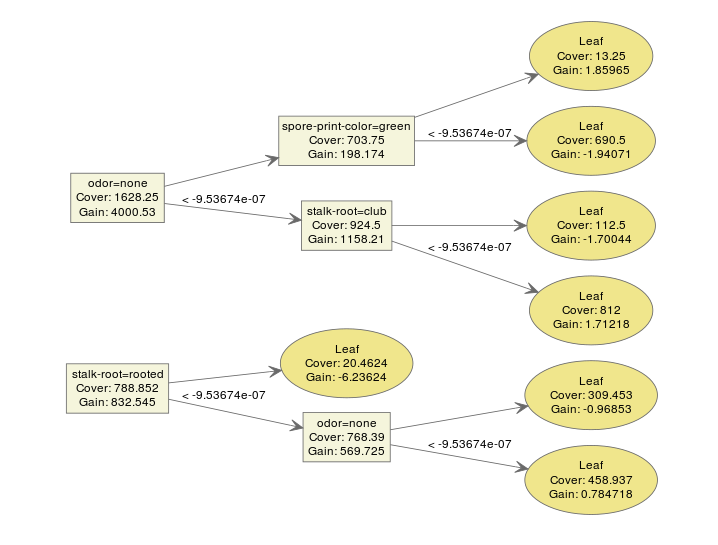We can make an interpretation on the model easily. `xgboost` provides a function `xgb.plot.tree` to plot the model so that we can have a direct impression on the result.

However, what if we have way more trees?

``````bst <- xgboost(data = train\$data, label = train\$label, max.depth = 2,
eta = 1, nthread = 2, nround = 10, objective = "binary:logistic")
xgb.plot.tree(feature_names = agaricus.train\$data@Dimnames[], model = bst)
``````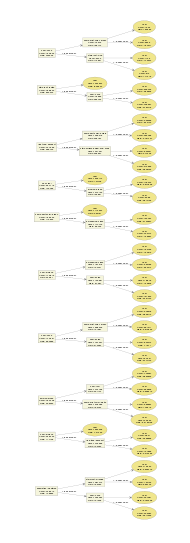It is starting to make things messy. We even have a hard time to inspect every detail on the plot. It is not easy to tell a story with too many conditions.

Multiple-in-one plot

In `xgboost`, we provide a function `xgb.plot.multi.trees` to ensemble several trees into a single one! This function is inspired by this blogpost: https://wellecks.wordpress.com/2015/02/21/peering-into-the-black-box-visualizing-lambdamart/. This is done with the following observations:

• Almost all the trees in an ensemble model have the same shape. If the maximum depth is determined, this holds for all the binary trees.
• On each node there would be more than one feature that have appeared on this position. But we can describe it by the frequency of each feature thus make a frequenct table.

Here is an example of an “ensembled” tree visualization.

``````bst <- xgboost(data = train\$data, label = train\$label, max.depth = 15,
eta = 1, nthread = 2, nround = 30, objective = "binary:logistic",
min_child_weight = 50)
xgb.plot.multi.trees(model = bst, feature_names = agaricus.train\$data@Dimnames[], features.keep = 3)
``````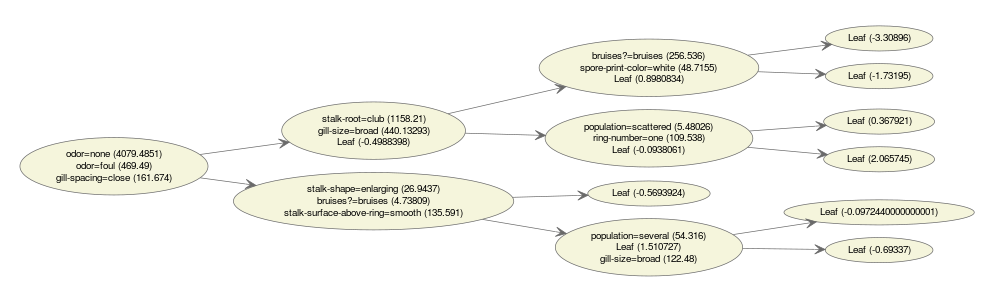The text in the nodes indicates the distribution of the features selected at this position. If we hover our mouse on the nodes, we get hte information of the path.

Feature Importance

If the tree is too deep, or the number of features is large, then it is still gonna be difficult to find any useful patterns. One simplified way is to check feature importance instead. How do we define feature importance in `xgboost`?

In `xgboost`, each split tries to find the best feature and splitting point to optimize the objective. We can calculate the gain on each node, and it is the contribution from the selected feature. In the end we look into all the trees, and sum up all the contribution for each feature and treat it as the importance. If the number of features is large, we can also do a clustering on features before we make the plot. Here’s an example of the feature importance plot from the function `xgb.plot.importance`:

``````bst <- xgboost(data = train\$data, label = train\$label, max.depth = 2,
eta = 1, nthread = 2, nround = 2,objective = "binary:logistic")
importance_matrix <- xgb.importance(agaricus.train\$data@Dimnames[], model = bst)
xgb.plot.importance(importance_matrix)
``````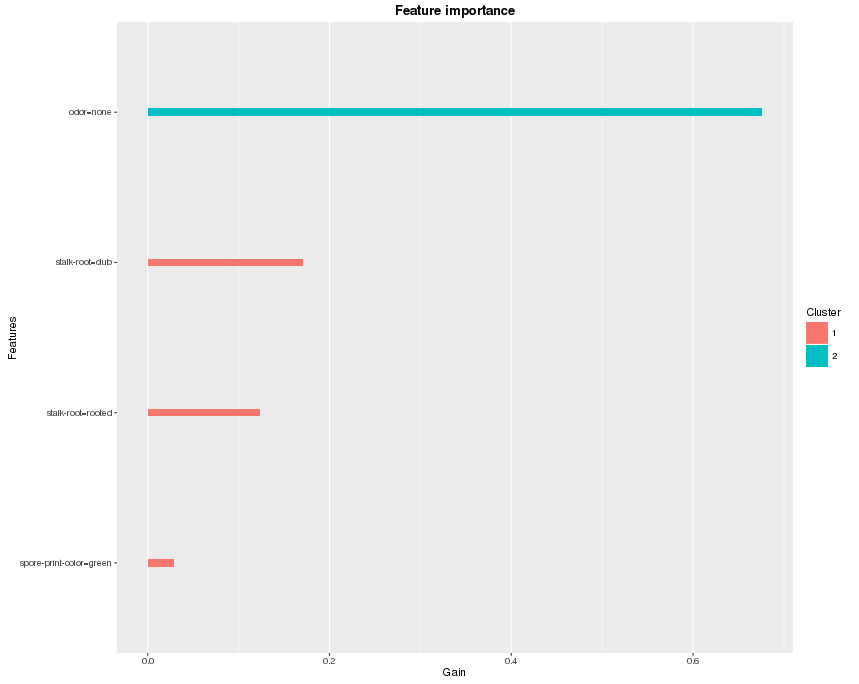Deepness

There is more than one way to understand the structure of the trees, besides plotting them all. Since there are all binary trees, we can have a clear figure in mind if we get to know the depth of each leaf. The function `xgb.plot.deepness` is inspired by this blogpost: http://aysent.github.io/2015/11/08/random-forest-leaf-visualization.html.

From the function `xgb.plot.deepness`, we can get two plots summarizing the distribution of leaves according to the change of depth in the tree.

``````bst <- xgboost(data = train\$data, label = train\$label, max.depth = 15,
eta = 1, nthread = 2, nround = 30, objective = "binary:logistic",
min_child_weight = 50)
xgb.plot.deepness(model = bst)
``````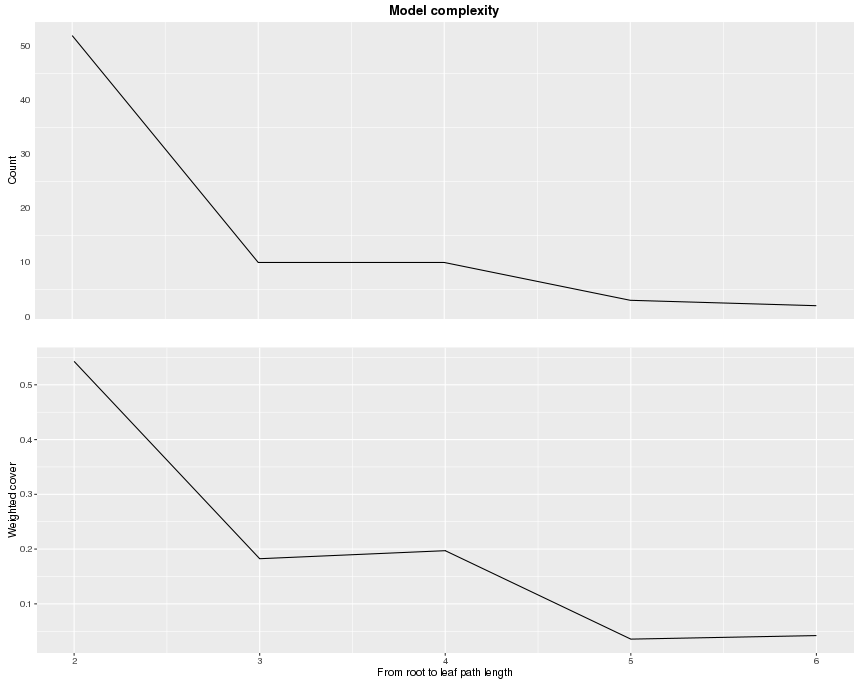The upper plot shows the number of leaves per level of deepness. The lower plot shows noramlized weighted cover per leaf (weighted sum of instances). From this information, we can see that for the 5-th and 6-th level, there are actually not many leaves. To avoid overfitting, we can restrict the depth of trees to be a small number.